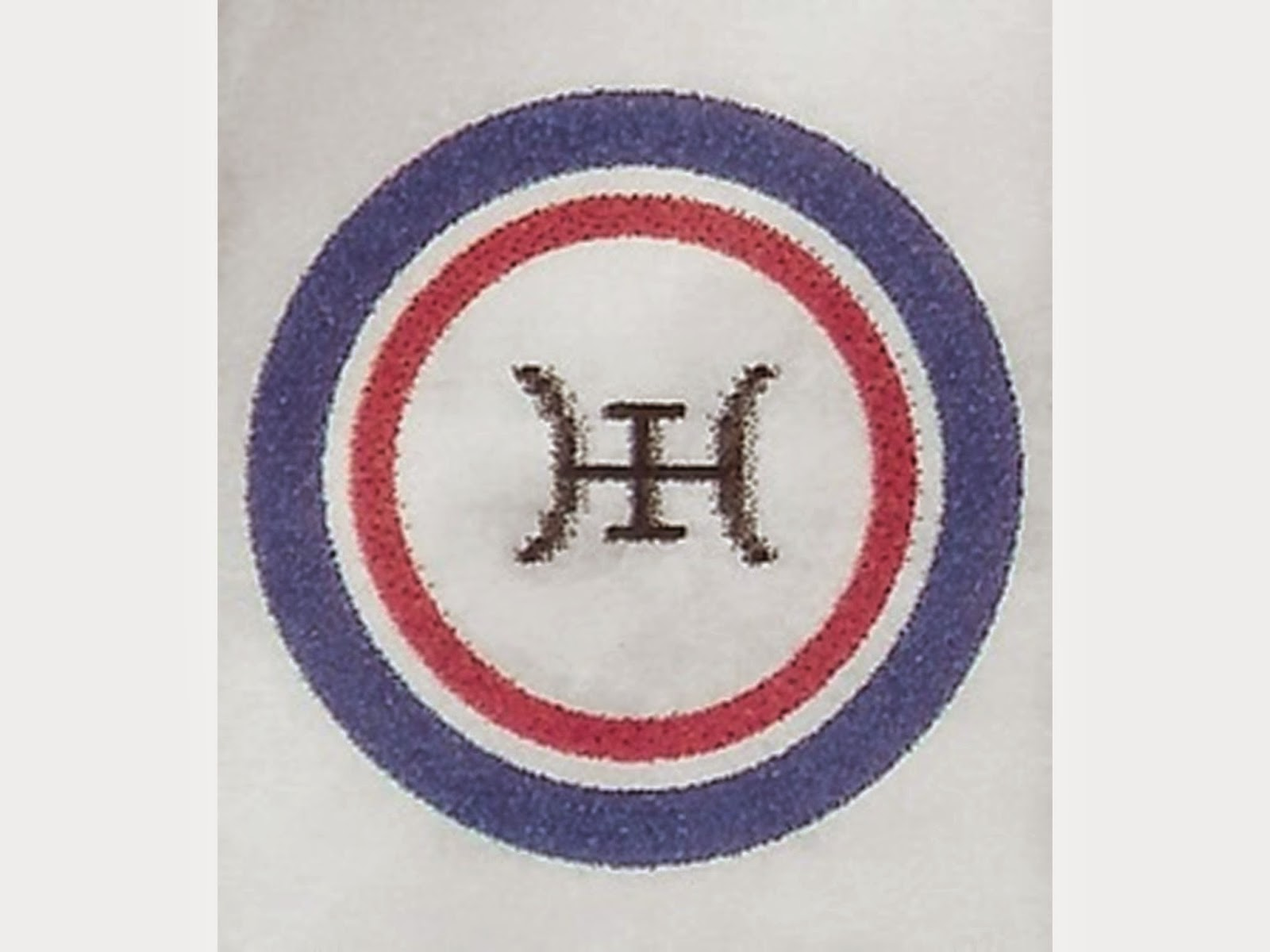## Featured Post

### First Interview with Karl Seldon, F.E.D. Co-Founder & Generalized-Equity Relation-of-Production Discoverer## Thursday, March 08, 2018

### “Introduction to the System of Dialectical Logic” -- Ivan Punchev’s 4-volume treatise.

“Introduction to the System of Dialectical Logic” -- Ivan Punchev’s 4-volume treatise.

# “Introduction to the system of dialectical logic

The work of Ivan Punchev entitled “Introduction to the system of dialectical logic” is composed by four books dedicated to one of the most fundamental and most difficult problems in philosophy.  This is building of integral system of rational dialectical logic. Currently such system exists (as a mystical type) only in Hegel, and in rational form this logic was applied only in Marx’s “Capital”.  Despite the efforts of many philosophers for more than a century, solution to this problem has not been achieved ​​entirely.  The problem is further complicated with the advent of the mathematical logic as a historic new stage in the development of formal logic.  This situation is related also with the famous “third crisis” in the foundations of mathematics started by the introduction of the mathematical concept of infinity in Set Theory and the consequently raised antinomies.  At the same time, the mathematical logic created a new standard for contemporary systematization of any scientific knowledge and thus put a requirement for mathematical modeling of the classic dialectical logic.  This issue is central to the four volumes.  In its context are studied problems of the history of the idea of a mathematical dialectical logic and dialectical mathematics. The scale and depth of this problematic situation require exploration of all main ideas in philosophy, logic and mathematics.  This determines the nature of this study as integrative, interdisciplinary and complex.”

I posted the following comment to this blog-entry, hoping to open a dialogue with the blog-entry’s author:

“It might be interesting to compare the work of Dr. Punchev with that of another project which has also derived a “new non-classical mathematical dialectics and dialectical mathematics”. In the case of this other project, their first “mathematics of dialectics” is both a ‘contra-Boolean’ algebra for dialectical logic, and a non-standard model of the Peano “Natural” numbers arithmetic, such as was foretold by both the Lowenheim-Skolem Theorem, and the “first order” logic co-application of the Goedel Completeness and Incompleteness Theorems. This work is available for free download via http://www.dialectics.org .”